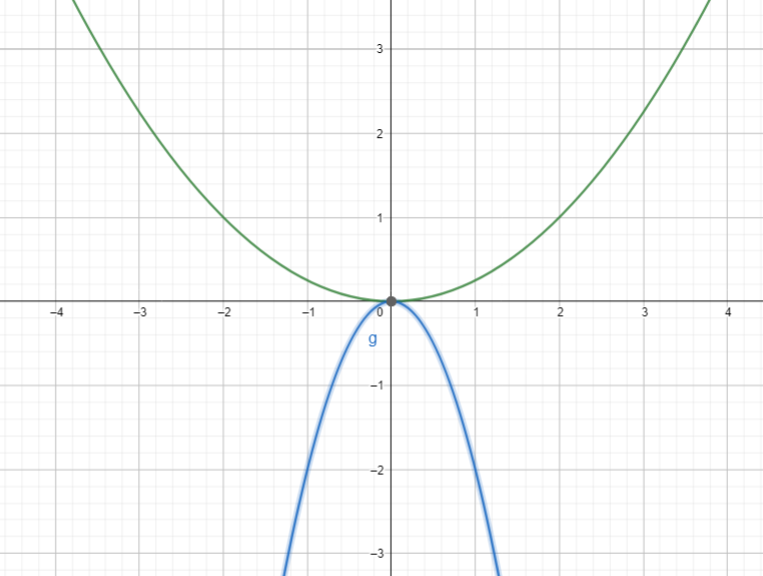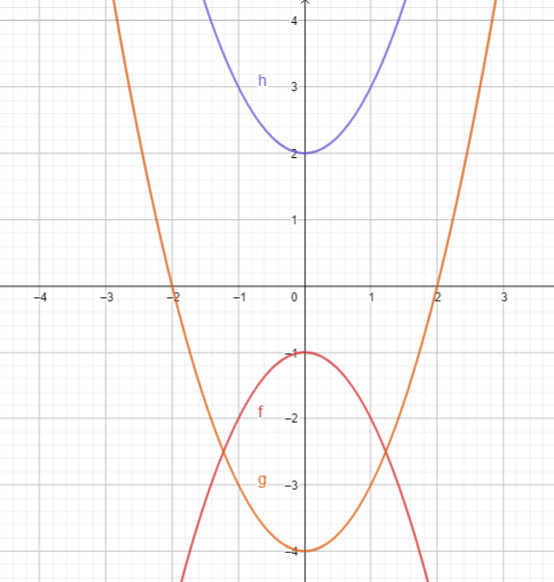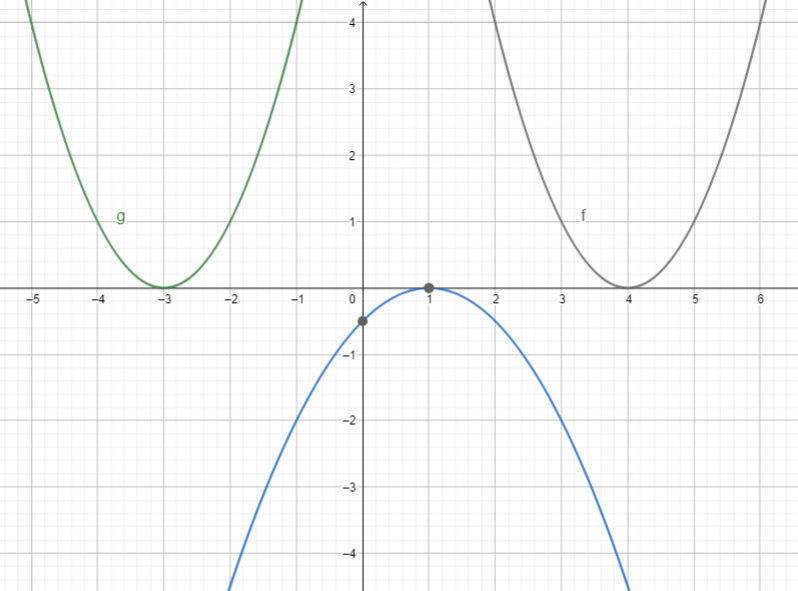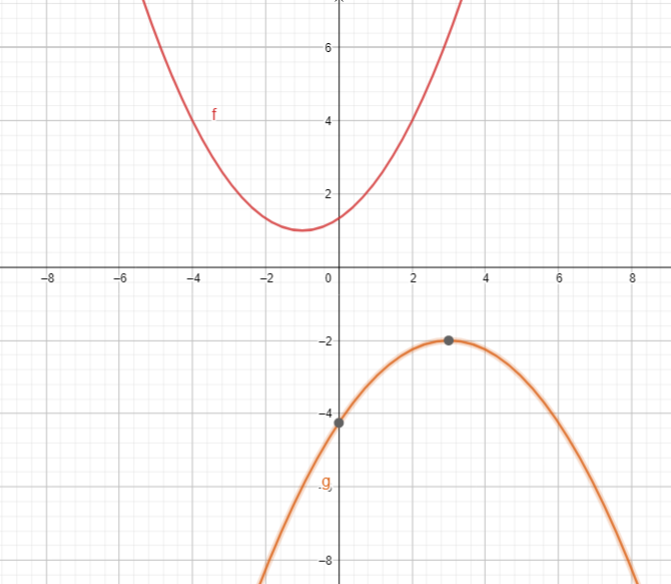# Algebra 1 - Quadratic Functions

## Introduction

• A quadratic function is a function whose defining equation can be expressed in the form .
• A quadratic function can be written in a standard form $\mathbit{y}\mathbf{=}\mathbit{a}{\mathbit{x}}^{\mathbf{2}}\mathbf{+}\mathbit{b}\mathbit{x}\mathbf{+}\mathbit{c}$, or the vertex form  are the coordinates of the vertex.
• The vertex $\left(h,k\right)$ can be solved by using the following formulas:

• The minimum or maximum value of a quadratic function is determined by using the formula $\mathbit{k}\mathbf{=}\frac{\mathbf{4}\mathbf{a}\mathbf{c}\mathbf{-}{\mathbf{b}}^{\mathbf{2}}}{\mathbf{4}\mathbf{a}}$. Another way is by directly substituting the value of $\mathbit{h}$ to the function and computing for the function value. The graphical method can also determine a quadratic function's minimum or maximum point.
• The graph of a quadratic function is a smooth u-shaped curve called a parabola.

The following are forms of a quadratic function:

1.

Example: Graph of2. . The graph of the function has a vertical shift. The parabola shifts upward if $\mathbit{k}\mathbf{>}\mathbf{0}$ or it shifts downward if $\mathbit{k}\mathbf{<}\mathbf{0}$. The vertex of this parabola lies either on the positive y-axis or the negative y-axis.

Example: Graph of3. . The graph of the function has a horizontal shift—the graph shifts to the left if $\mathbit{h}\mathbf{<}\mathbf{0}$ or right when $\mathbit{h}\mathbf{>}\mathbf{0}$.

Example: Graph of4. . The vertex form exactly indicates the transformation of the graph. The $\mathbit{h}$ units indicate a horizontal shift while $\mathbit{k}$ units indicate the vertical shift of the parabola.

Example: Graph of## Properties of Quadratic Function and its Graph

The properties of the quadratic function and its graph are as follows:

• The parabola opens upward if $\mathbit{a}\mathbf{>}\mathbf{0}$. It opens downward if $\mathbit{a}\mathbf{<}\mathbf{0}$.
• The vertex $\left(h,k\right)$ is the lowest/minimum point if the parabola opens upward.
• The vertex $\left(h,k\right)$ is the highest/maximum point if the parabola opens downward.
• The domain of quadratic functions is the set of all real numbers.
• The range depends on the value of $\mathbit{k}$ in the vertex $\left(h,k\right)$ and its direction of opening:  if it opens upward, and if it opens downward.
• The line or axis of symmetry is the line that separates the parabola into two halves or regions.
• A parabola represented by a quadratic function has only one y-intercept.
• The zeros of the quadratic function can be solved by letting $y=0$. Common methods of solving for zeros are the same as solving for the roots of quadratic equations. These are factoring, extraction of roots, completing the square, using the Po-Shen Loh method, or using the quadratic formula.
• A parabola may have two x-intercepts (when there are two real distinct zeros), one x-intercept/tangent to the x-axis (when there is only one zero), or no x-intercept (when zeros are not real).
• The parabola gets narrower or closer to the line of symmetry when the absolute value of $\mathbit{a}$ increases. It gets wider or moves away from the line of symmetry when the absolute value decreases.

## Methods of Deriving the Equation of Quadratic Function

The quadratic function equation can be derived given the following information: table of values, the graph, some points on the graph, and the zeros.

Steps in sketching the graph of a quadratic function:

• Find the coordinates of the vertex by solving for the x-value using the formula $\mathbit{x}\mathbf{=}\mathbit{h}\mathbf{=}\mathbf{-}\frac{\mathbf{b}}{\mathbf{2}\mathbf{a}}$. Then, direct substitute the obtained x-value to the quadratic function and solve for the y-coordinate of the vertex. The formula $\mathbit{k}\mathbf{=}\frac{\mathbf{4}\mathbf{a}\mathbf{c}\mathbf{-}{\mathbf{b}}^{\mathbf{2}}}{\mathbf{4}\mathbf{a}}$ can also be used to find the y-coordinate.
• Construct a table of values using at least four points (consisting of two x-values greater than the x-coordinate of the vertex and two x-values less than the x-coordinate of the vertex).
• Sketch the graph by plotting the points and the vertex. Then, illustrate the graph by connecting the points, making a smooth curve.

## Cheat Sheet

• Always inspect the sign of  in the equation of the quadratic function. These values tell the direction of the parabola's opening, shape, and translation.
• If $|a|\mathbf{<}\mathbf{1}$, the graph is wider than the graph of $\mathbit{f}\left(x\right)\mathbf{=}{\mathbit{x}}^{\mathbf{2}}\mathbf{.}$.
• If $|a|\mathbf{>}\mathbf{1}$, the graph is narrower than the graph of $\mathbit{f}\left(x\right)\mathbf{=}{\mathbit{x}}^{\mathbf{2}}\mathbf{.}$
• $\mathbit{f}\left(x\right)\mathbf{=}\frac{\mathbf{1}}{\mathbf{8}}{\left(x-2\right)}^{\mathbf{2}}\mathbf{+}\mathbf{2}$ has a wider graph unlike the graph of $\mathbit{g}\left(x\right)\mathbf{=}\mathbf{5}{\left(x-2\right)}^{\mathbf{2}}\mathbf{+}\mathbf{2}$.
• The parabola $\mathbit{f}\left(x\right)\mathbf{=}\mathbf{2}{\left(x+1\right)}^{\mathbf{2}}\mathbf{-}\mathbf{1}$ has a vertex at $\left(-1,-1\right)$.
• To change the standard equation of the parabola , another option is Completing the Square.
• Quadratic functions have the same properties as Absolute Value Functions except for the shape of their graphs.

## Blunder Areas

• A quadratic function differs from a quadratic equation since the former involves independent and dependent variables while the latter consists of only the independent variable.
• A quadratic function whose graph does not touch the x-axis has no real zeros.
• A quadratic function is an example of a not one-to-one function. Hence, its inverse is also not one-to-one.